SIMION8 FAQHome   Important information IP Partners Institutions Teachers Accepted Students Rules CPOTS 2013 Venue Dates Proposal Summary Participant Rules Group photo Participant photosPhoto Gallery CPOTS2013 Course outlineDetailed Work ProgrammeFor Teachers For Students CPOTS2013 Official student evaluation formCPOTS2013 Private evaluation formLow res poster (jpg - 1.8MB) High res poster (jpg - 17.5MB) Course Material For All registered CPOTS 2013 participants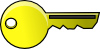Students Final Grades SIMION projects SIMION SIMION FAQ SIMION ASMS courses Subsistence & Travel Social Program Sponsors   Useful Links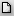CPOTS NEWSCharged Particl Optics: Theory and Simulation
Project coordinator: Prof. Theo Zouros,
Dept. of Physics, University of Crete

This project has been funded with support
from the European Commission

Home > SIMION FAQ

# SIMION 8 FAQ for CPOTS

New GEM file commands (extensions in v. 8.2) and syntax
Excel VBA Reference

SIMION user programming flow-chart (from Christoph Lemell's Advanced SIMION lecture AS2-3).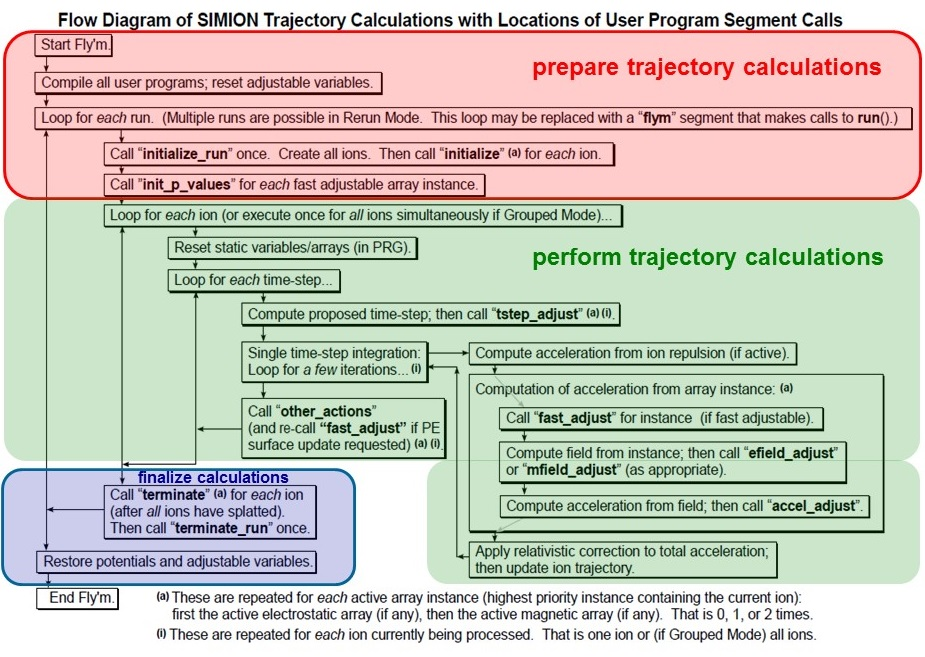A more recent update (March 2017) can be seen below: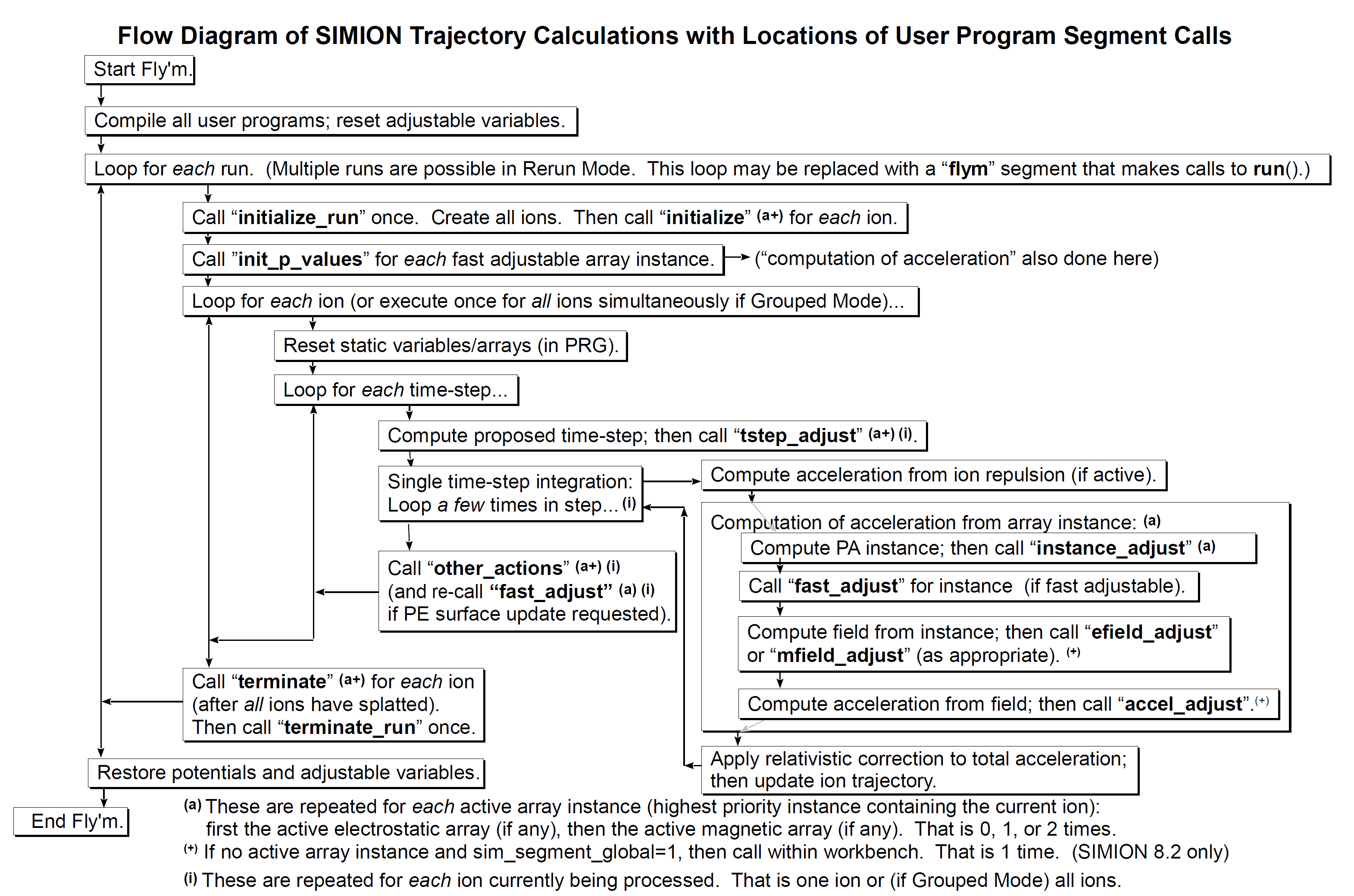Example of how a user defined adjustable parameter obtains its value:

1. You click Fly'm.
2. The top-level of the Lua program is run, which defines adjustable variables (e.g. "adjustable value = 1" ) and defines segments.
3. Values from the Variables tab are copied to/from the adjustables in the program. So, if for example you set "value" to 3 from the Variables menu, it gets set in the program here.
4. Next segments get run. Let's say now your segment initialize_run sets "value = 2" here. Since step 4 always overrides step 3 "value=2" from here on until the whole Fly'm is executed.

SIMION IOB coordinate system and angular conventions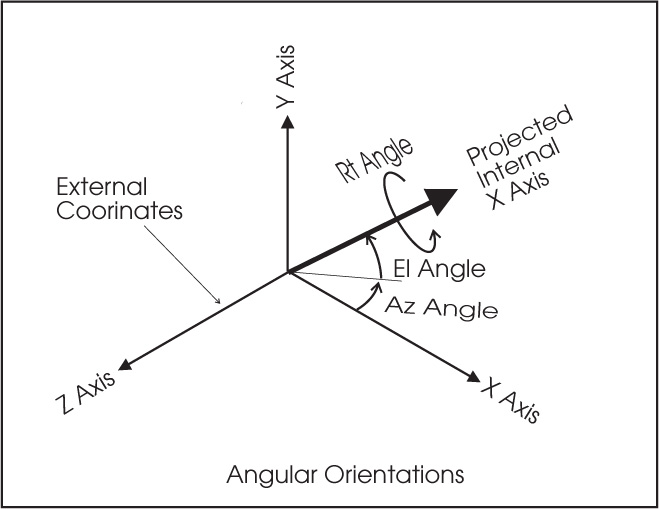From immediate inspection of the above figure we get for a general vector A (Ax,Ay,Az):
Ax = A cos El cos Az
Ay = A sin El
Az= -A cos El sin Az

From which we can in general compute angles El and Az as:

tan Az = -Az/Ax,

tan El = Ay/sqrt(Ax^2+Az^2)

The values of A, Az and El can be computed by the following lua code rect3d_to_polar3d_rad (x,y,z) where in general (x,y,z) can be replaced by (Ax,Ay,Az)

How to obtain angles Az and El from position vector r (x,y,z)

```-- similar to the simion function but in radians
local r = math.sqrt(x*x + y*y + z*z)
local az
if z ~= 0 or x ~= 0 then
az = math.atan2(-z, x)
else
az = 0
end
local r2 = math.sqrt(x*x + z*z)
local el
if y ~= 0 or r2 ~= 0 then
el = math.atan2(y, r2)
else
el = 0
end

return r, az, el
end
```

Useful SIMION tricks and other hard to find info.

Examples of parametrized gem files:

See SIMION Exaples:
• examples/einzel/einzel_param.gem
• examples/spectrum/ppa2d_slits.gem
• examples/field_emission/fe_geom.gem
• examples/geometry_optimization/mesh_size.gem
• examples/geometry/sphere_aniso.gem
• examples/kingdon_trap/ kingdon_2dc.gem
• examples/kingdon_trap/twocylinder_1dc.gem

How to draw/markout a line or point in View (can be used to show a reference line such as test plane or other or just place a mark at some important reference point)

simion.experimental.plot_line_segment( x1,y1,z1, x2,y2,z2, v1, v2, color1, color2, mark)

This function plots a 3D line segment using the same mechanism that SIMION uses to draw trajectories. The given line segment is immediately drawn on the View screen GUI (if trajectory viewing or GUI mode is not suppressed) and/or written to any trajectory file (trj\*.tmp or .kept_traj) for future re-display (if trajectory retaining is not suppressed). You may use this function to plot trajectories calculated outside of SIMION or simply to draw lines on the screen (applications include drawing custom field/contour lines and drawing boundaries of otherwise invisible objects like gas flow arrays).

The line segment is plotted from point ``(x1,y1,z1)`` to point ``(x2,y2,z2)`` in workbench coordinates (mm). The two points are also given potentials ``v1`` and ``v2`` respectively (used only for PE surface views, in the vertical axis).

The two points are also given color indices ``color1`` and ``color2`` respectively. If trajectories are drawn as lines (not dots), then the line is drawn with color ``color1``. If trajectories are drawn as dots, then during the animation point 1, which is assumed to have been previously drawn with color ``color1``, is erased and point 2 is then drawn with color ``color2``. (The erasing is done with "XOR" painting, which will not fully erase if ``color1`` is not correctly specified.)

If ``mark`` is ``true``, then a marker is drawn at the first point. The marker is drawn using the global marker color (which is set via the color in Time Markers on Particles tab in View screen and cannot currently be changed programatically). A marker color of 15 takes special meaning: the marker is drawn using the line color (``color1``).

``x1,y1,z1, x2,y2,z2, v1, v2`` are real numbers. Presently they must be finite (not +-math.huge or 0/0). color1, color2 are integers from 0 (black) to 15 (transparent). mark is a boolean (true, false).

``x2,y2,z2`` if omitted (nil) default to ``x1,y1,z1``. ``v2`` if omitted (``nil``) defaults to ``v1``. ``color2`` if omitted (nil) defaults to ``color1``. ``mark`` if omitted (``nil``) defaults to ``false``, unless if ``x1,y2,z2`` are also omitted, in which case it defaults to ``true``.

Note: ``plot_line_segment(x1,y1,z1)`` will plot a marker at the given point.

GUI Library (simion.experimental.dialog)

See link at GUI Library (simion.experimental.dialog)

Differences in the way electrodes are displayed in Modiy and View screens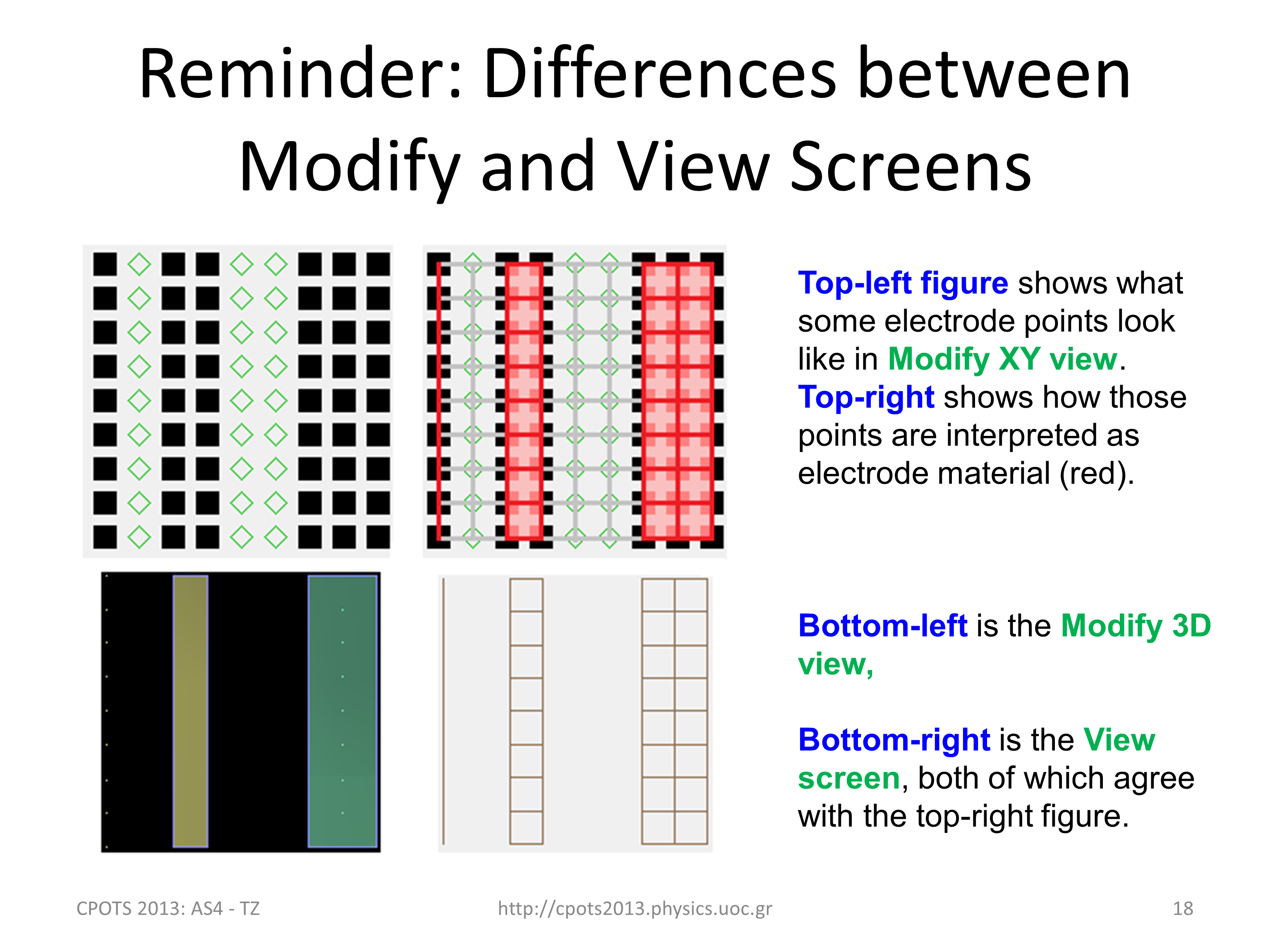Define Pa with surface=fractional

```# d_mm = 0.1         -- mm/gu
# local W = 51	     	-- mm width of plates
# local Lx = 258.6    -- mm length in x direciton
# local Ly = 114.6    -- mm length in y direction
# local Lz = W/2	      -- half of width of ppa's (W/2 - see ppa1, ppa2)

# local nx = round(Lx/d_mm)+1
# local ny = round(Ly/d_mm)+1
# local nz = round(Lz/d_mm)+1

pa_define(\$(nx),\$(ny),\$(nz), planar,surface=fractional,z, electrostatic,, \$(d_mm))
```

Above example using parametrization. d_mm gives the grid density in mm/gu.

With surface=fractional, voltages defined on any electrode points are taken as precise boundary conditions to Laplace. Furthermore, red bars near electrode points assume the potentials of the nearby electrode points. That doesn't quite do for infinitely thin electrodes between grid points. As a workaround, you can make the electrode have finite thickness (0-1 gu maybe), and if it only overlaps a single row of grid points, it will allow particles to pass through in Flym (presently Flym ignores red bars and only looks at electrode points), but then fields in only one side of the electrode would be highly accurate.There's nothing preventing Refine from doing this better; it's just a matter of representing it in the PA.

How to make the editor opening lua files in SIMION to use a particular user defined editor (e.g. SCITE) in Win 7

Define a new Win 7 Enviroment variable SIMION_EDITOR and set it equal to the path of the desired exe, e.g.
SIMION_EDITOR = C:\Program Files\SIMION-8.1\simion-editor-20121229\SciTE.exe

To define the Environment variable in Win 7 follow directions at: http://www.itechtalk.com/thread3595.html

To run lua code (e.g. The lua program in a file named test.lua) from the SIMION command bar:

Type in the command bar the following:
`dofile 'test.lua'`
Make sure the file test.lua is in the active directory

To activate a new update in the Simion directories:

For example if you want the new version of Statistics.lua to become active [after placing it in the appropriate SIMION directory (..\lib\lua\simionx)], you have to restart SIMION or enter this into the SIMION command bar:
`package.loaded['simionx.Statistics'] = nil`

Using the lua SC editor tab to indent blocks of code

Highlight the block of code and then press either TAB or SHIFT+TAB. And Options|Change Indentation Settings controls tab style.

How to ensure 1gu transparency (mesh) in a variable density gem file

Just make the mmgu a variable and use it for the width, more of less like this:
```# local mmgu = 0.1  -- mm per grid unit
pa_define(............., \$(mmgu))
e(1) { fill { within { box2d(\$(10-mmgu/2),0, \$(10+mmgu/2),5) } } }```

Example to load a particular fly (fly2, ion etc) file from lua rather than have to do it manually?

The simion.experimental.add_particles is the 8.2 early access function that does something like this. It's demonstrated in examples\child_particles (latest version). However, this defines a FLY2 definitions within the current Lua file not from an external FLY2 file.

Save the whole trajectory screen automatically to some file via lua at the end of the fly?

See examples\geometry_optimize\tune.lua

``` -- Print image of screen to file.
simion.printer.type = 'png'  -- 'bmp', 'png', 'jpg'
simion.printer.filename = 'output/result_' .. dx .. '.png'
simion.printer.scale = 1
simion.print_screen()```
dx is any variable that differentiates one result from the other. Make sure the "output" directory exists.

How to define a Gaussian distribution in fly2 file:

```   f=simion.fly2.gaussian_distribution{mean=0,stdev=1}
for i=1,20 do print(f()) end```

Using the iterator method to define a distribution in fly2 file:

```local position = simion.fly2.circle_distribution {center =
simion.fly2.vector(82.55, 0, 28, normal = simion.fly2.vector(0, 0, -1),

local p = position()
print(p, p, p)
```

How to define a Lorentzian distribution in fly2 file:

It can be done by editing the FLY2 in a text editor:

```-- http://en.wikipedia.org/wiki/Cauchy_distribution
local function lorentz(mu, phi)
return distribution(function()
return mu + phi*math.tan(math.pi*(rand() - 0.5))
end)
end

particles {
standard_beam {
n = 100,
mass = 10, charge = -1,
ke = lorentz(100, 1)
}
}```

Greek characters in Lua for use in Excel plot titles and other

Unicode characters can be inserted by specifying their UTF-8 byte encoding, as shown below (for γ, which has Unicode U+hex notation U+03B3 and UTF-8 code unit byte encoding hexidecimal "CE B3"). One tool to obtain the "UTF-8 code units" for a character is http://rishida.net/tools/conversion/ .

The Windows Character Map (charmap.exe) program can help in finding Unicode symbols with U_hex notation.

See the following common examples:

```local C_alpha = string.char(0xCE, 0xB1)  --α
local C_beta = string.char(0xCE, 0xB2)  --β
local C_gamma = string.char(0xCE, 0xB3)  -- γ
local C_Alpha = string.char(0xCE, 0x91)  -- Α
local C_Beta = string.char(0xCE, 0x92)  -- Β
local C_Gamma = string.char(0xCE, 0x93)  --Γ
local C_Delta = string.char(0xCE, 0x94)  -- Δ
local C_plusminus = string.char(0xC2, 0xB1)   -- ±
local C_deg = string.char(0xC2, 0xB0) -- °
```

Different color markers for different fly groups in the same fly:

In the Particle tab - Trajectory display change the color time marker to 15 (white). Then the markers use the color set for the trajectories in the Define initial fly parameters.

Regenerate PA's if dimensions in GEM have changed:

The Lua command regenerate() can be given either via a lua program or by giving this command in the command line of SIMION. This is useful in the case you have modified a gem file and then want to save it and refine it. The regenerate() command does exactly that. It works only in the View screen environment not Modify.

Potential Array (PA) Scaling in GEM files

PA size

It's 8 bytes on disk and 10 bytes in RAM.

At each point in the PA, the potential is stored using a double precision floating point value, which is 8 bytes. In addition SIMION needs to store whether that point is an electrode or non-electrode. Sometimes it stores that 1 bit of information within the same double precision floating point value. At other times it is more efficient to store it separately. SIMION maintains an extra 2 byte integer for this purposes and other purposes (e.g. when refining).

Page F-7 in the manual shows the format in which PA's are stored on disk. The pa files are NOT ASCII files!

Selectively refining a single pa without opening the SIMION gui

Give the following command to refine electrode number 11 with convergence criterion 1E-7 from the simion.exe directory speficying the path where the example pa (here tanden.pa#) lies.

simion.exe --nogui lua -e "local pa = simion.pas:open('simion.exe --nogui lua -e "local pa = simion.pas:open('D:/SIMION/tandem_d_mm=0.1/tandem.pa#');pa:refine{solutions={11}, convergence=1e-7}"

to also crop right after:

simion.exe --nogui lua -e "local pa = simion.pas:open('D:/SIMION/tandem_d_mm=0.1/tandem.pa#');
pa:refine{solutions={11}, convergence=1e-7};
pa:crop(0,0,0, 99,10,0);
pa:save()"

Cropping pas to cut down on RAM usage

Cropping a pa via Modify function in SIMION

With the pa (.pa0 or .pa) already refined in SIMION you may go into the Modify function and use the cursor to define cropping regions in the 3-D gu volume. Make sure you also save the original uncropped pas for future use.
If you crop the .pa0 then this will automatically also crop the associated .pa_i (i=1,2,3,...)

Cropping a pa via lua control

```simion.pas:close() 		-- equivalent to button Remove All PAs from RAM - clears all pas before the next command.
local pa = simion.pas:open('HDA.pa0')		-- loads the pa0
pa:crop(0,0,0, 1270,71,1150, true)		-- crops - see the crop command in simion manual for more details
```

The above 3-line lua code can be placed in a file with extension .lua (for example crop.lua) and run from SIMION by name from the SIMION button "Run Lua Program"

You may also crop without opening the SIMION gui:

local pa = simion.pas:open('drag.pa#')
pa:refine{solutions={0}, convergence=1e-7}
pa:crop(0,0,0, 99,10,0)
pa:save()

Adding a la carte contours of potential or electric fields via lua and with different colors

See example below:

```simion.wb.contours = {
potentials = {
{value=100.0; color=1};
{value=110.0; color=1};
{value=120.0; color=5};
};
{value=20.0; color=2};
{value=30.0; color=2};
};
}```

Change the size of the marker:

Use the "Size" field on the Trajectory Display panel on Particles tab to increase the marker size.

Screen dump of particular SIMION window view only: Put cursor on window of interest click and then hit

ALT+PrintScr

Lua control of electrode voltages in different instances in the workbench:

Answer: use the ion_instance reserved variable to differentiate electrodes with same number but in different instances. For example:

```simion.workbench_program()
if ion_instance == 1 then
elseif ion_instance == 2 then
end
end```

Lua control of active instance in the workbench:

There's a new "instance_adjust" segment, which allows you to re-order PA instance priorities during a Fly'm. Example:

```simion.workbench_program()
if ion_instance == 2 then
if ion_px_mm > 10 then
ion_instance == 0  -- suppress
end
end
end```

That causes PA instance #2 to be invisible in the region x > 10 mm, effectively allowing particles to instead see any fields from any lower PA instances (i.e. #1). This can be useful in examples like "bender_cut" where a system is modeled by two PA's that partly overlap in the case when the boundary conditions at the end of the arrays might not be realistic--you can cut-off the end of those arrays. One way to do that is with the "Crop" function on the PA itself. This instance_adjust segment provides an alternate approach which doesn't touch the PA file and is more flexible in defining the shape of the region to mask out. Yet another way to achieve this is by overriding the efield_adjust segment as shown in the first link below, but that is can be less clean.

Further details at:

It is available in SIMION 8.2 early access 20170214 or above (previewable in 8.1.3.0-TEST on Check for Updates). This segment can be useful for systems with multiple PA's that partly overlap.

To add lua code in gem files:

Example of if statement in gem file:
```# if x == 1 then
e(1) { fill { within { circle(0,0,10) } } }
# else
e(2) { fill { within { circle(0,0,10) } } }
# end```
You can even define your own functions for reusable shapes.... Something like
```# function mybox(x,y)
e(1) { fill { within { box(\$(x),\$(y),\$(x+1),\$(y+1)) } } }
# end

# for i=1,10 do
\$(mybox(i*10,0))
# end
```
http://simion.com/info/gem_geometry_file.html mentions some of this but not all possible usage.

Lua control of Display and Rerun in Particle Menu:

sim_retain_trajectories (which controls both Retain and Display of trajectories), but you could just do "sim_rerun_flym = 1" at the top of the flym segment which also has that effect.

For Loops in Lua:

Use only integers in for loop or do this:
```for gloop=g_start,g_end+g_step/2,g_step do
... code ...
end```

Histogramming/binning routine in lua:

The "simionx.Statistics" library (see Help file) computes a histogram (i.e. binning). examples\plot\tof_histogram.lua uses it.

Lua code to print out values of potential at fixed z=0 and variable radius and angle:

```-- test.lua
--local pa = simion.wb.instances.pa
local pa = simion.pas
local z = 0
for r=0,10,2 do
local s = ""
for t=0,math.pi, math.pi/16 do
local x = math.cos(t) * r
local y = math.sin(t) * r
local v = pa:potential_vc(x,y,z)
s = s .. "\t" .. v
end
print(s)
end```

To compare values of SIMION pa and theory:

In the main (opening menu page of SIMION) Remove All PAs from RAM and load the ".pa0" file of your pa.

Then enter the following into the bottom SIMION command bar:

pa=simion.pas; for y=30,60 do print(y, pa:potential(0,y,0), 0+((-600)-(0))/ln(60/30) * ln(y/30)) end

The output you get will look like this:

```30        0        0
31        -28.380277755053        -28.383428867014
32        -55.859687813145        -55.865642634889
33        -82.493553548814        -82.502114249961
34        -108.33263087424        -108.34334738509
35        -133.42271068667        -133.43545280187
36        -157.80638552688        -157.82064350028
37        -181.52196344606        -181.53766201226
38        -204.60553522397        -204.62215070104
39        -227.0894767889        -227.10697395224
40        -249.00461463579        -249.02249956731
41        -270.37858466928        -270.39684540574
42        -291.23789048036        -291.25609630215
43        -311.60634322853        -311.62449545615
44        -331.50687545511        -331.52461381727
45        -350.96016568088        -350.97750043269
46        -369.98617924867        -370.0028162691
47        -388.602998686        -388.61895364147
48        -406.82810883686        -406.84314306758
49        -424.67741562398        -424.69154910401
50        -442.16631694961        -442.17935649972
51        -459.30888047795        -459.32084781779
52        -476.11873190155        -476.12947351954
53        -492.60837663564        -492.61791537281
54        -508.78992512088        -508.79814393297
55        -524.67455014521        -524.68147074968
56        -540.27305388165        -540.27859586945
57        -555.59546808616        -555.59965113373
58        -570.65145973968        -570.65423971143
59        -585.45007909758        -585.45147225199
60        -600        -600```

This is a more direct way to evaluate the accuracy (without any particle tracing) by compare the potentials in the PA against the theoretical equation. This is a lua command and prints a table for the values of y=30 to 60 of the pa (0,y,0) entries and compares them to the theoretical result computed in the next column. Small differences between SIMION (left column) and theory (right column) are indicative of SIMIONs accuracy in this example. This a quick trick to compare simulation with theory and very useful in establishing the simulation accuracy of SIMIONs solution to the Laplace equation. The example is from a cylindrical mirror analyzer with R1=30 and R2=60 with V(R1)=0 and V(R2)=-600V having its y-axis along the interradial distance (see http://simion.com/info/cma.html ).

Please send in your "discovery" as it will probably be useful to all.

 Last Update: 01/05/17 00:48:02 Home        Web Support        Contact        Emergency        FAQ